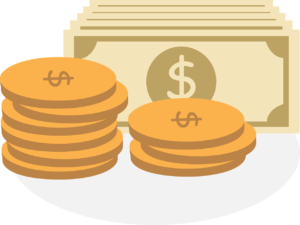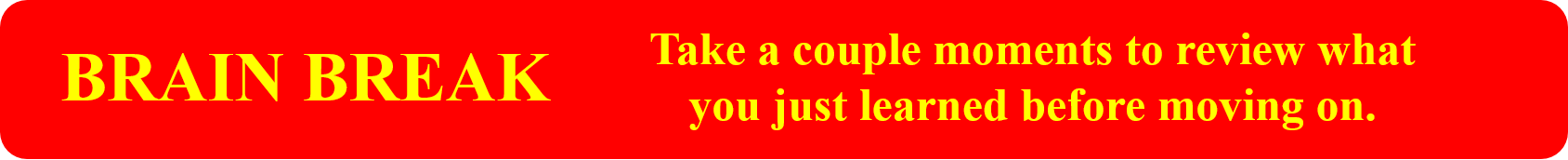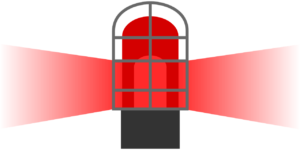Percentages

# Expressing Change as a Percent

Melissa is a carpentry foundation student who has just finished her six-month foundation program. This is a program for people who are looking to get into the trade but are not yet apprentices.It’s now time to make some money after spending the previous six months in school. After Melissa graduated from the program, she applied for three jobs and decided to take the one that offered the best value. Her starting wage is $14 per hour, and after six months, it will move up to$16 per hour.

This is a $2 per hour pay raise, but does this represent a good percentage increase? If she started at$25 per hour and went up to \$27 per hour, would this be the same percentage increase?

The answer lies in the following formula:

$\text{Percentage change}=\dfrac{\text{Actual increase or decrease}}{\text{Original amount}}\times100$

There are a couple of things to note here. One is that this formula works if you have either an increase or a decrease from the original amount. If Melissa’s wage went down, you could also express that as a percentage. The second thing to note is that there is the number 100 at the end of the formula. This is due to the fact that the calculation would give us a decimal, and multiplying this number by 100 makes it a percentage.

So, to follow through on our calculation, let’s put the numbers in and see what we get.

$\LARGE\text{Percentage change}=\dfrac{\2}{\14}\times100$

$\LARGE\text{Percentage change}=0.1429\times100$

$\LARGE\text{Percentage change}=14.29\%$

This is telling us that Melissa will get a 14.29% increase in her wage after the first six months. Not bad!

ExampleDuring her foundation program, Melissa had to keep her job working at a coffee shop in order to pay the bills. On her first day of work, she served 78 cups of coffee. During her six months on the job, the most cups of coffee she made in one day was 201. How can the difference between these amounts be expressed as a percentage increase?

Step 1: Calculate the increase in cups served.

$\LARGE201-78=\text{123 more cups of coffee}$

Step 2: Write down the formula you are going to work with.

$\text{Percentage change}=\dfrac{\text{Actual increase or decrease}}{\text{Original amount}}\times100$

Step 3: Plug the numbers into the formula.

$\LARGE\text{Percentage change}=\dfrac{123}{78}\times100$

Step 4: Work through the answer.

$\LARGE\text{Percentage change}=1.58\times100$

$\LARGE\text{Percentage change}=158\%$

This answer tells us that, from the time Melissa started to the time she had her most productive day, she had a one-day increase of 158%. If she were to increase her output by 100%, that would mean mathematically that she would be making twice as much coffee as before. Increasing by 158% indicates that she is making MORE than twice the amount of coffee as when she first started.

# Practice Questions

Answer the questions below and then check the video answers to see how you did.

Question 1

In its first year of operation, a trade school has 57 students. In its second year of operation, its student body increased to 104 students. What percent increase is this?

Question 2

Referring back to the trade school in question 1, their third year of operation did not go so well. In their third year, the number of students went down to 86. What percent decrease is this from year two?# Calculating Percentage Changes

The company Melissa works for currently has 27 apprentices working, and they want to increase that number in the coming year. In the previous section, we would have asked how many more apprentices they were looking to hire. But what if the goal was to increase the number of apprentices by 20%? How would you go about doing that?

So what we have is this:We have to incorporate percent increase to find the number of apprentices the company wants to have in the end. The company currently has 27 apprentices and is looking to increase that number by 20% in the next year. The first thing we need to find out is what 20% of 27 is. Remember that 20% is like 20 out of 100. We can use this concept to find our answer.

$\LARGE\dfrac{20}{100}=0.2$

Multiply 27 by 0.2 to get our increase.

$\LARGE27\times0.2=5.4$

What this answer indicates is that the company wants to increase their number of apprentices by 5.4. Now, we can’t have 0.4 of an apprentice, so we have to round up or down. Following the rules we established before, we round down to 5.

In the end, the company wants to increase the number of apprentices by 5. But we are not quite done yet: we still have to calculate how many apprentices they want to have in the future. To do this, we simply add the 5 extra students to our starting number, and we end up with 32 students.

$\LARGE27+5=32$

ExampleWe’re going to change it up here and use sports as our example.

A professional hockey team scored 219 goals in the 2018–2019 season and finished in 26th place in the league. In the 2019–2020 season, they want to improve on that total and are looking for a 25% increase in goal production. How many goals would they have to score to achieve their goal?

Step 1: Calculate 25% of 219.

$\LARGE\dfrac{25}{100}=0.25\text{, so }219\times0.25=54.75$

As we can’t score 0.75 of a goal, we need to round this up to 1 complete goal and make it 55 more goals.

Step 2: Add 55 to our original number, and we get:

$\LARGE219+55=274\text{ goals}$In the end, to achieve the 25% increase in goal production that they are looking for, the team would have to score 274 goals. If they scored that many in the 2018–2019 season, they would end up in the top 5 or 6 teams for goal production.

# Practice Questions

Answer the following questions and check the video answers to see how you did.

Question 1

Melissa, the carpentry apprentice, likes to garden during the spring and summer. This year, she planted 42 plants. Next year, she wants to increase the total number of plants by 15%. How many plants will she need to plant in order to achieve her goal?

Question 2

Let’s go back to sports for this one. A quarterback threw 47 touchdown passes in one year. The next year, the number of touchdown passes he threw dropped by 35%. How many touchdown passes did he throw that year?